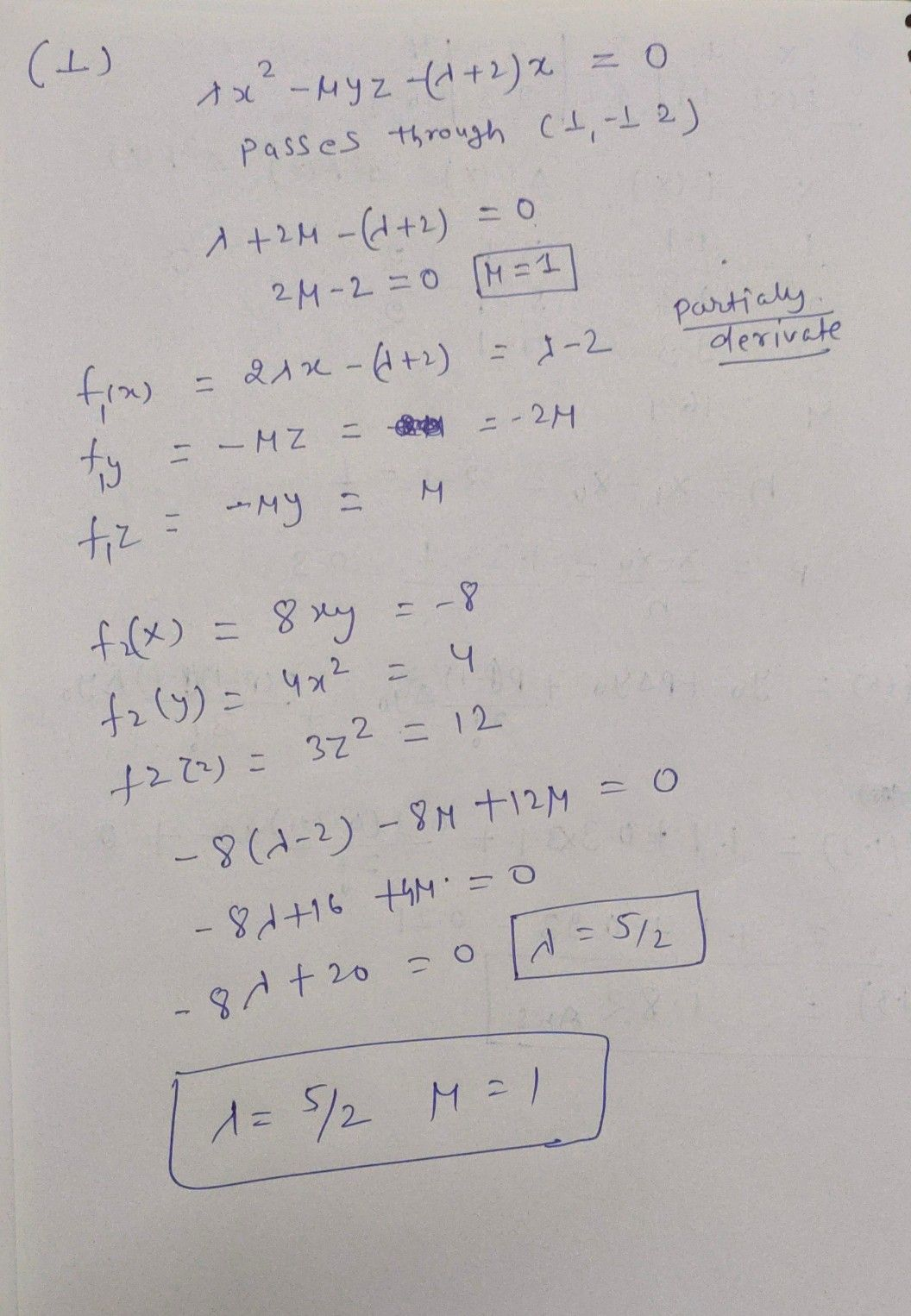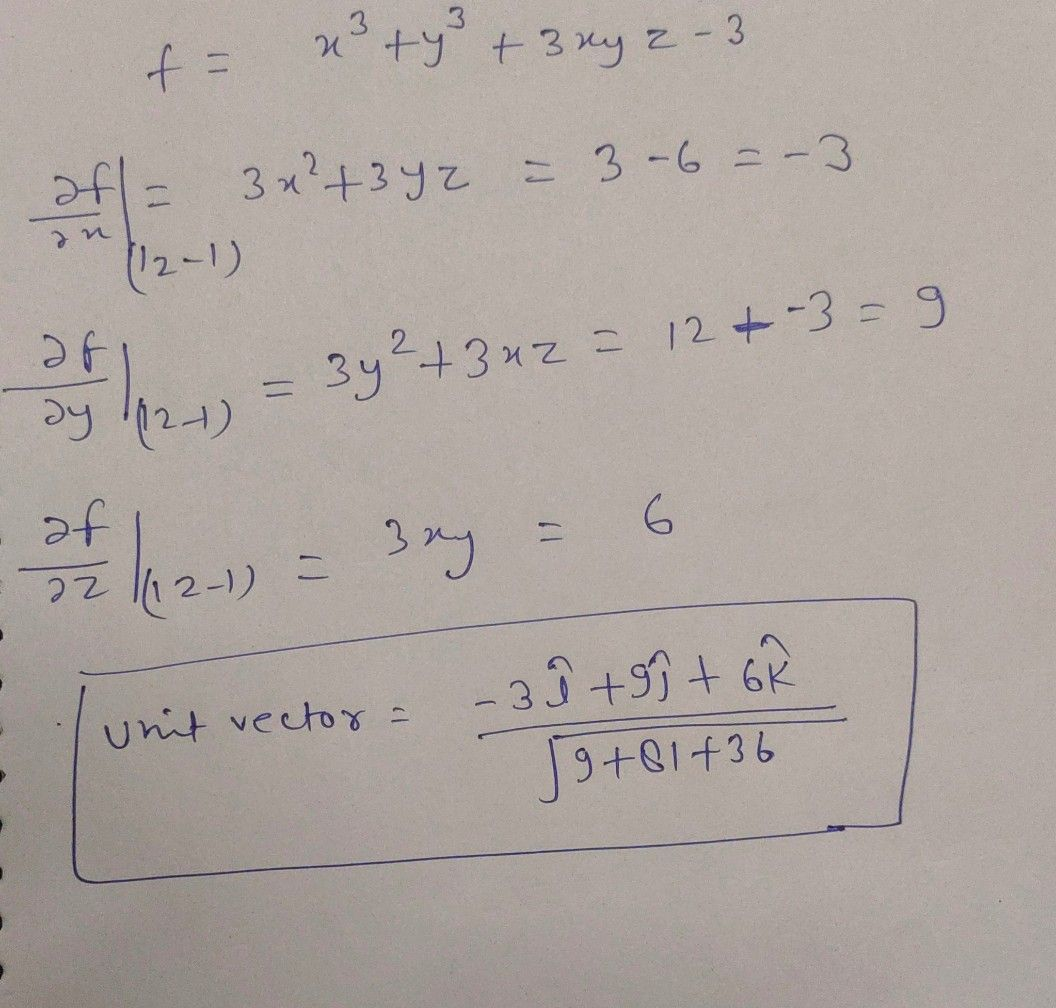Symbol
ProblemVector Calculus $1.$ Find the values of constants A and u so that the surfaces $Ax^{2}-yz=\left(x+2\right)x$ $and$ $4x^{2}y+z^{3}=4$ intersect orthogonally at the point $\left(1,-1,2\right)$ 2. Find unit vector normal to the $\bar{5}$ $x^{3}+y^{3}+3xyz=3$ at the point $\left(1,2,-1\right)$ 3. Verify Green's theorem for $\int _{c}\left(\left(xy+y^{2}\right)dx+x^{2}dy\right)$ where $C$ is the boundary of $y=x$ and $y=x^{2}$ 4. Using Stokes' theorem, evaluate $\int _{c}\left(\left(x+y\right)dx+\left(2x-z\right)dy+\left(y+z\right)dz\right)$ where C is the boundary of the triangle with $orticos$ $\left(2,0,0\right),\left(0,3,0\right),$ $\left(0,0,6\right)$ taken over the region in the first 5. Verify divergence theorem for $\vec{A} =2x^{2}yi-y^{2}i+4xz^{2}k$ $exy^{2}+z^{2}=9andthcp$ plane $x=2$ octant bounded by the cylinder
Calculus
Search count: 108
SolutionQanda teacher - PALIWAL
which questionStudent
can u 2nd question sirQanda teacher - PALIWAL
I have already sent 2 second question see second pictureStudent
i wont to this all solution sir asap
pleaseQanda teacher - PALIWAL
at a time 1question is allowed